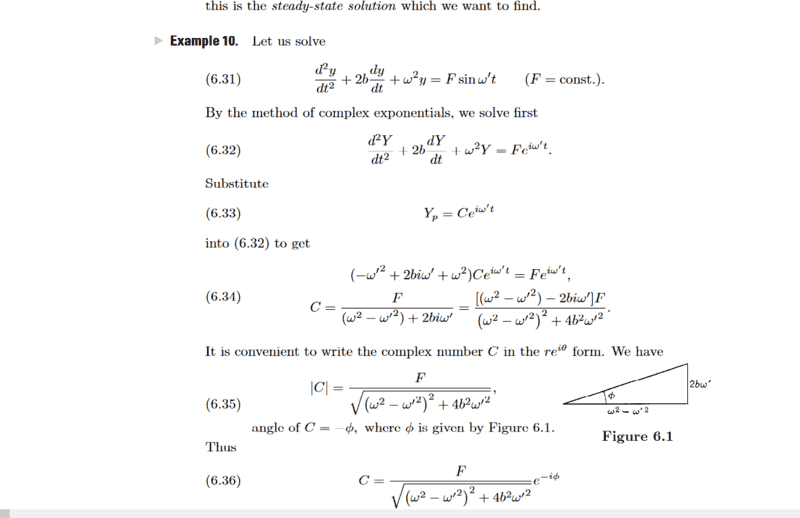# Need Help with this Step in a DE solution

• I
• bigmike94

#### bigmike94

TL;DR Summary
There's a step in this book which i am not sure i follow
at the bottom where it says equation 6.35, the magnitude of C, how do we get from equation 6.34 to 6.35? I separated the fraction into 2, one with a real part and the other imaginary and tried taking the magnitude then and didn't come up with the same as in the book. Can someone help show me what I am missing, please?#### Attachments

This is simply the complex norm: ##C=\dfrac{1}{x+iy}=\dfrac{x-iy}{(x+iy)(x-iy)}\Rightarrow |C|=\dfrac{1}{\sqrt{x^2+y^2}}=r.##

•bigmike94